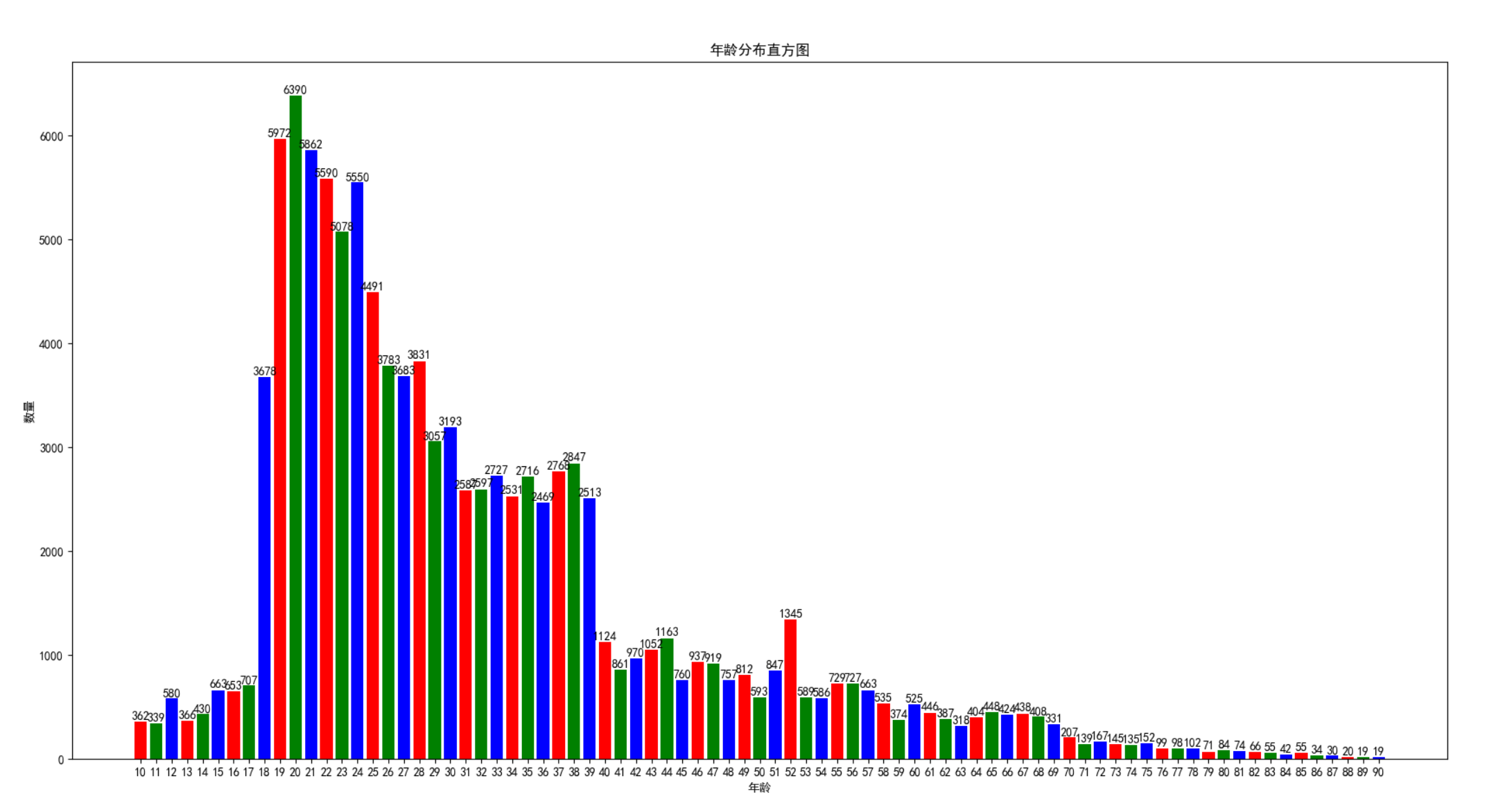## 基于MXNET实现的年龄性别识别# 环境

• 安装 mxnet，支持 1.3~1.6 版本，安装命令如下。
``````pip install mxnet-cu101==1.5.0
``````

# 数据集

• 默认支持以下三种数据集，将以下三个数据集下载解压到 `dataset` 目录下。
2. http://mmlab.ie.cuhk.edu.hk/projects/MegaAge/
3. https://ibug.doc.ic.ac.uk/resources/agedb/
• 执行生成数据列表。
``````python create_dataset.py
``````
• 如果想训练自定义数据集，只需生成类似以下的数据列表就可以了。
``````dataset/AgeDB/0_MariaCallas_35_f.jpg,0,35
dataset/AgeDB/10000_GlennClose_62_f.jpg,0,62
dataset/AgeDB/10001_GoldieHawn_23_f.jpg,0,23
dataset/AgeDB/10003_GoldieHawn_24_f.jpg,0,24
dataset/AgeDB/10004_GoldieHawn_27_f.jpg,0,27
dataset/AgeDB/10005_GoldieHawn_28_f.jpg,0,28
dataset/AgeDB/10006_GoldieHawn_29_f.jpg,0,29
``````# 训练

``````python train.py
``````

``````gpu num: 1
num_layers 50
data_shape [3, 112, 112]
Called with argument: Namespace(batch_size=128, color=0, ctx_num=1, cutoff=0, data_dir='dataset', data_shape='3,112,112', end_epoch=200, gpu_ids='0', image_channel=3, image_h=112, image_w=112, lr=0.1, lr_steps='10,30,80,150,200', network='m50', num_layers=50, prefix='temp/model', pretrained='', rand_mirror=1, rescale_threshold=0, version_input=1, version_output='GAP')
1 GAP 32
INFO:root:dataset\train.rec 数据大小：303018
INFO:root:是否随机翻转图片：1
INFO:root:dataset\val.rec 数据大小：1032
INFO:root:是否随机翻转图片：False
call reset()

INFO:root:Epoch Batch [0-20]	Speed: 520.85 samples/sec	acc=0.572545	MAE=10.734747	CUM_5=0.240699
INFO:root:Epoch Batch [20-40]	Speed: 518.95 samples/sec	acc=0.589844	MAE=9.351172	CUM_5=0.289844
INFO:root:Epoch Batch [40-60]	Speed: 516.86 samples/sec	acc=0.603125	MAE=9.184766	CUM_5=0.303906
INFO:root:Epoch Batch [60-80]	Speed: 508.44 samples/sec	acc=0.609766	MAE=8.759375	CUM_5=0.336719
INFO:root:Epoch Batch [80-100]	Speed: 461.26 samples/sec	acc=0.656250	MAE=8.224609	CUM_5=0.361328
INFO:root:Epoch Batch [100-120]	Speed: 518.43 samples/sec	acc=0.696875	MAE=7.611328	CUM_5=0.400391
INFO:root:Epoch Batch [120-140]	Speed: 514.88 samples/sec	acc=0.715234	MAE=7.224609	CUM_5=0.426172
INFO:root:Epoch Batch [140-160]	Speed: 517.80 samples/sec	acc=0.722266	MAE=6.976172	CUM_5=0.437500
``````

# 评估

``````python eval.py
``````

``````100%|██████████| 1032/1032 [00:06<00:00, 153.75it/s]

``````

# 预测

``````python infer.py --image=test.jpg
``````

``````第1张人脸，位置(160, 32, 204, 84), 性别：男, 年龄：30

``````